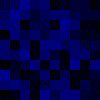Sean McLemon | Advent of Code

2021-12-11 - Dumbo Octopus

(original .ipynb)

Day 11 puzzle input is a grid of integers representing "energy level" of dumbo octopuses (mine is here). Over discrete time steps their energy increments, and if at any time it exceeds 9 then this increases to neighbouring octopuses' energy levels also (and if they exceed 9 this gives a boost to neighbouring octopuses and so on, it cascades). Part 1 involves finding how many times an octopus crossed that energy level from 9 to 10 (i.e. it "flashes") over 100 time steps. Part 2 involves finding the number of time steps that pass before all octopuses "flash" in one particular step.

test_input_str = """5483143223
2745854711
5264556173
6141336146
6357385478
4167524645
2176841721
6882881134
4846848554
5283751526"""

def parse_input_lines(input_str):
return [
[int(c) for c in line]
for line
in input_str.split("\n")
]

def increase_neighbours(grid, r, c, flashed):
neighbours = neighbours8(grid, r, c)
for nr, nc in neighbours:
grid[nr][nc] += 1
if grid[nr][nc] > 9 and not ((nr,nc) in flashed):
increase_neighbours(grid, nr, nc, flashed)

flashed = set()

for r, row in enumerate(grid):
for c, col in enumerate(row):
grid[r][c] += 1
if grid[r][c] > 9 and not ((r,c) in flashed):
increase_neighbours(grid, r, c, flashed)

return flashed

def part_one(input_str, steps=100):
grid = parse_input_lines(input_str)
total_flashes = 0

while steps > 0:
total_flashes += len(flashed)
for r, c in flashed:
grid[r][c] = 0
steps -= 1

assert 1656 == part_one(test_input_str)
print("part one:", part_one(puzzle_input_str))
part one: 1571

palette = {n:f"#00{hex(n)[2:]}" for n in range(10)}
palette = "#fff"

def part_two(input_str, show_image=True):
grid = parse_input_lines(input_str)
total_octopuses = len(grid) * len(grid)
step = 0
flashed = set()
image_frames = [grid_animated_render_frame(grid, palette)]

while len(flashed) != total_octopuses:
for r, c in flashed:
grid[r][c] = 0
step += 1
image_frames.append(
grid_animated_render_frame(grid, palette)
)

grid_animated_combine(image_frames, "day11-p2.gif", show=show_image)

return step

assert 195 == part_two(test_input_str, False)
print("part two:", part_two(puzzle_input_str))part two: 387